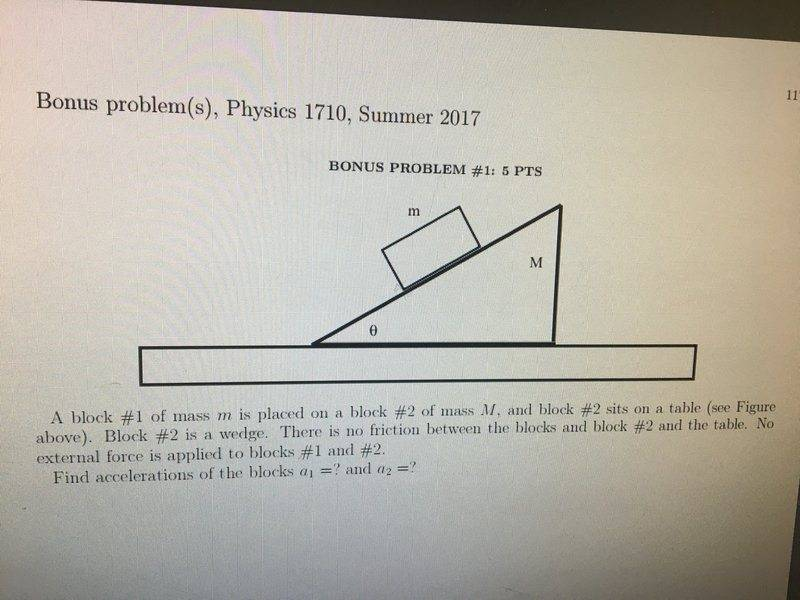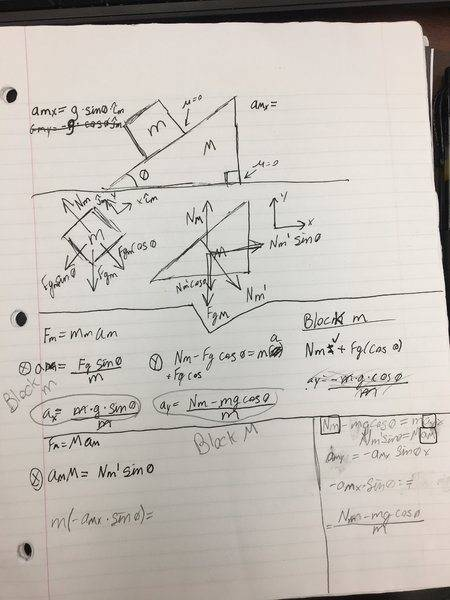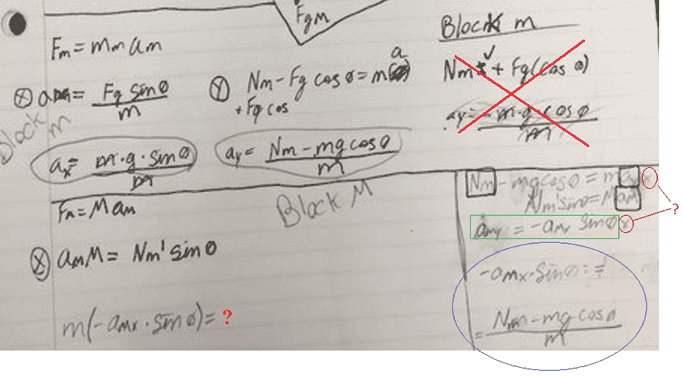# Acceleration of a frictionless block on a frictionless wedge

## Homework StatementThere is no friction between any surfaces mass is known. Find acceleration of the blocks.

F=ma

## The Attempt at a SolutionI'm almost certian
Amx=g sin θ
AMy=0

Hmm.... Why wouldn't the acceleration in the x direction be affected by the mass of the objects?

•haruspex
TSny
Homework Helper
Gold Member
Amx=g sin θ
AMy=0
Yes, these look correct.

Most of your work looks OK to me.The part x-ed out in red is incorrect, but it doesn't look like you used that part.

Look at your final equation circled in blue. Can you see a substitution that you can make for ##N_m## in this equation that would allow you to solve for the acceleration of the wedge, ##a_{Mx}##?

One of the key equations for this problem is the constraint ##a_{my} = -a_{Mx} \sin \theta## (boxed in green). But I don't see where you showed how you obtained this.

haruspex
Homework Helper
Gold Member
Amx=g sin θ
That would be true if the wedge were fixed. But imagine if the wedge were not only frictionless but extremely light. The block would be almost in free fall.

TSny
Homework Helper
Gold Member
That would be true if the wedge were fixed. But imagine if the wedge were not only frictionless but extremely light. The block would be almost in free fall.
For the block, the x direction is parallel to the incline. So, free fall acceleration would have an x-component of magnitude g sinθ.

haruspex
Homework Helper
Gold Member
For the block, the x direction is parallel to the incline. So, free fall acceleration would have an x-component of magnitude g sinθ.
I had not picked up that x was defined as parallel to the slope.

•person123
phinds